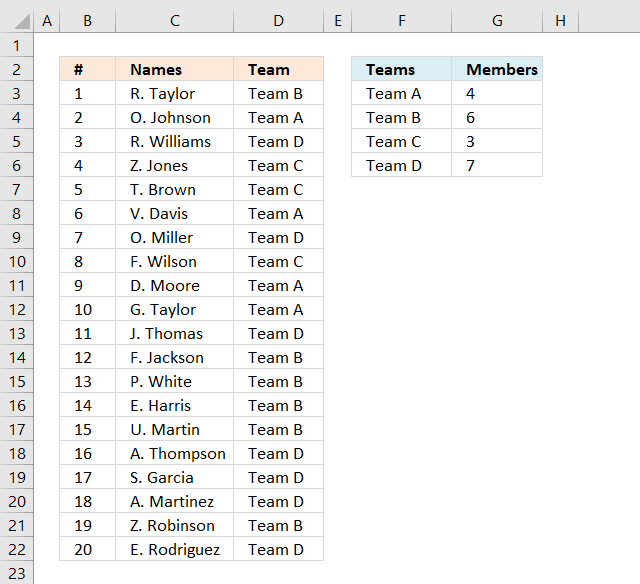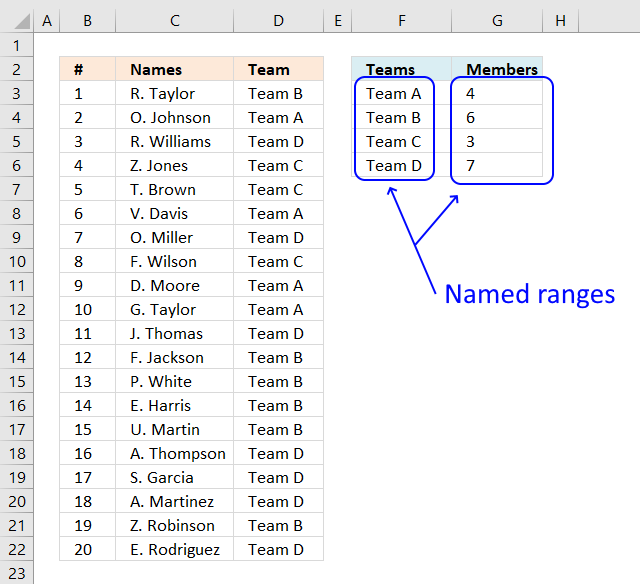Author: Oscar Cronquist Article last updated on April 26, 2021JD asks in this post: Dynamic team generator
Hi, what if we have different number of people per team? So in team A, there could be a max of 4 members but in team B there could be a max of 6 members etc. Is there some formula which can be used for this as well?

Yes, there is. This array formula uses the values in cell range D2:E4 to randomly assign people to teams, demonstrated in column B and C.

## 1. Team Generator (Formula)The image above shows a formula in column D that assigns teams to names randomly based on the number of team members specified in column G.

Change the team names in column F if you like and type a number in the corresponding cell in column G to force the formula to assign a maximum number of team members.

Array formula in cell D3:

=IFERROR(INDEX(Teams, LARGE((COUNTIF(\$D\$2:D2, Teams)<>Members)*MATCH(ROW(Teams), ROW(Teams)), RANDBETWEEN(1, SUM(--(COUNTIF(\$D\$2:D2, Teams)<>Members))))), "")

This array formula contains a couple of excel functions, if you want to know more about them check out these posts: LARGE, INDEX, COUNTIF, IFERROR, MATCH, ROW, RANDBETWEEN. It also has both absolute and relative cell references, learn more.

## 2. How to enter an array formula

1. Copy above array formula
2. Select cell C2
3. Paste array formula to the formula bar
4. Press and hold CTRL + SHIFT simultaneously
5. Press Enter once

If you did it right the formula now begins and ends with curly brackets, like this {=array formula}. Don't enter the curly brackets yourself.

## 3. How to copy the formula to cells below

1. Select cell C2
2. Copy cell (Shortcut key CTRL + c)
3. Select cell range C3:C21
4. Paste (Shortcut key CTRL + v)

You can also select cell C2, press and hold on the black dot on the lower right corner of cell C2. Drag it down as far as needed.

## 4. Named ranges in the array formulaThere are two named ranges in this worksheet, Teams and Members. They return a cell range in column F and G respectively that grow automatically as you add new values.

### 4.1 How to create a named range

1. Copy formula below.
2. Go to tab Formula on the ribbon.
3. Press with left mouse button on the "Name Manager" button. A dialog box appears.
4. Press with left mouse button on the "New..." button located on the dialog box. Another dialog box appears.
5. Paste formula to "Refers to;" field.
6. Name the named range in "Name:" field.
7. Press with left mouse button on OK button.
8. Repeat steps 4 to 7 to create the second named range.
9. Press with left mouse button on OK button on the first dialog box to apply changes and dismiss it.

Teams:

=Sheet1!\$F\$3:INDEX(Sheet1!\$F\$3:\$F\$101,COUNTA(Sheet1!\$F\$3:\$F\$101))

Members:

=Sheet1!\$G\$3:INDEX(Sheet1!\$G\$3:\$G\$101,COUNTA(Sheet1!\$F\$3:\$F\$101))

## 5. Explaining formula in cell D3

IFERROR(INDEX(Teams, LARGE((COUNTIF(\$D\$2:D2, Teams)<>Members)*MATCH(ROW(Teams), ROW(Teams)), RANDBETWEEN(1, SUM(--(COUNTIF(\$D\$2:D2, Teams)<>Members))))), "")

#### Step 1 - Count previous teams in cells above

The COUNTIF function calculates the number of cells that are equal to a condition.

COUNTIF(rangecriteria)

The first argument is a cell reference that contains both a relative and absolute part meaning the cell ref grows when cell is copied to cells below.

The second argument is a dynamic named range meaning it grows when more values are added.

COUNTIF(\$D\$2:D2, Teams)

becomes

COUNTIF("Team", {"Team A"; "Team B"; "Team C"; "Team D"})

and returns {0; 0; 0; 0}.

#### Step 2 - Check if value is not equal to the total number of members in a team

The less than and larger than character combined means not equal to. This step makes sure that there is a correct number of assigned team members.

COUNTIF(\$D\$2:D2, Teams)<>Members

becomes

{0;0;0;0}<>Members

becomes

{0;0;0;0}<>{4;6;3;7}

and returns {TRUE; TRUE; TRUE; TRUE}

#### Step 3 - Convert boolean values to numerical

This step converts TRUE to 1 and FALSE to 0 (zero).

--(COUNTIF(\$D\$2:D2, Teams)<>Members)

becomes

--({TRUE; TRUE; TRUE; TRUE})

and returns {1; 1; 1; 1}. This step is needed to be able to calculate a sum.

#### Step 4 - Calculate total

The SUM function adds numbers from a cell range or an array and returns a total. It can't, however,  sum boolean values.

SUM(--(COUNTIF(\$D\$2:D2, Teams)<>Members))

becomes

SUM({1; 1; 1; 1})

and returns 4.

#### Step 5 - Create a random number based on Team member count

The RANDBETWEEN function returns a random whole number between the numbers you specify.

RANDBETWEEN(bottom, top)

RANDBETWEEN(1, SUM(--(COUNTIF(\$D\$2:D2, Teams)<>Members))))

becomes

RANDBETWEEN(1, 4)

and returns a pseudo-random number from 1 to 4.

#### Step 6 - Create an array from 1 to n

The ROW function returns the row number from a cell reference, if a cell range is used all numbers are returned in an array.

MATCH(ROW(Teams), ROW(Teams))

becomes

MATCH({3;4;5;6}, {3;4;5;6})

The MATCH function finds the relative position of a given value in a cell range or array. This step makes sure the array begins with 1.

MATCH({3;4;5;6}, {3;4;5;6})

returns {1;2;3;4}.

#### Step 7 - Extract k-th largest value

The LARGE function extracts the k-th largest number from an array or cell range.

LARGE(array, k)

LARGE((COUNTIF(\$D\$2:D2, Teams)<>Members)*MATCH(ROW(Teams), ROW(Teams)), RANDBETWEEN(1, SUM(--(COUNTIF(\$D\$2:D2, Teams)<>Members))))

becomes

LARGE((COUNTIF(\$D\$2:D2, Teams)<>Members)*MATCH(ROW(Teams), ROW(Teams)), 3)

becomes

LARGE(({TRUE; TRUE; TRUE; TRUE})*MATCH(ROW(Teams), ROW(Teams)), 3)

becomes

LARGE(({TRUE; TRUE; TRUE; TRUE})*{1;2;3;4}, 3)

becomes

LARGE(({TRUE; TRUE; TRUE; TRUE})*{1;2;3;4}, 3)

becomes

LARGE({1;2;3;4}, 3)

and returns 2.

#### Step 8 - Get value

The INDEX function returns a value from a cell range based on a row and column number (optional).

INDEX(Teams, LARGE((COUNTIF(\$D\$2:D2, Teams)<>Members)*MATCH(ROW(Teams), ROW(Teams)), RANDBETWEEN(1, SUM(--(COUNTIF(\$D\$2:D2, Teams)<>Members)))))

becomes

INDEX(Teams, 2)

becomes

INDEX({"Team A";"Team B";"Team C";"Team D"}, 2)

and returns "Team B" in cell D3.

#### Step 9 - Check if value is an error

The IFERROR function handles errors, you can specify what value to returns if an error is found.

IFERROR(valuevalue_if_error)

IFERROR(INDEX(\$F\$3:\$F\$19, LARGE((COUNTIF(\$D\$2:D2, Teams)<>Members)*MATCH(ROW(Teams), ROW(Teams)), RANDBETWEEN(1, SUM(--(COUNTIF(\$D\$2:D2, Teams)<>Members))))), "")

becomes

IFERROR("Team B", "")

and returns "Team B".

## 6. Disable automatic recalculation

The RANDBETWEEN function is volatile and each time you enter a new value somewhere on this sheet, array formulas are recalculated.

To prevent this from happening the following event code turns off automatic calculation. Activating sheet1 enables manual calculation, deactivating sheet1 enables automatic calculation.

```Private Sub Worksheet_Activate()
Application.Calculation = xlCalculationManual
End Sub
Private Sub Worksheet_Deactivate()
Application.Calculation = xlCalculationAutomatic
End Sub
```

Where is this code? Press with right mouse button on on tab sheet1. Press with mouse on "View Code..."

Remember to press F9 after editing teams and member columns.